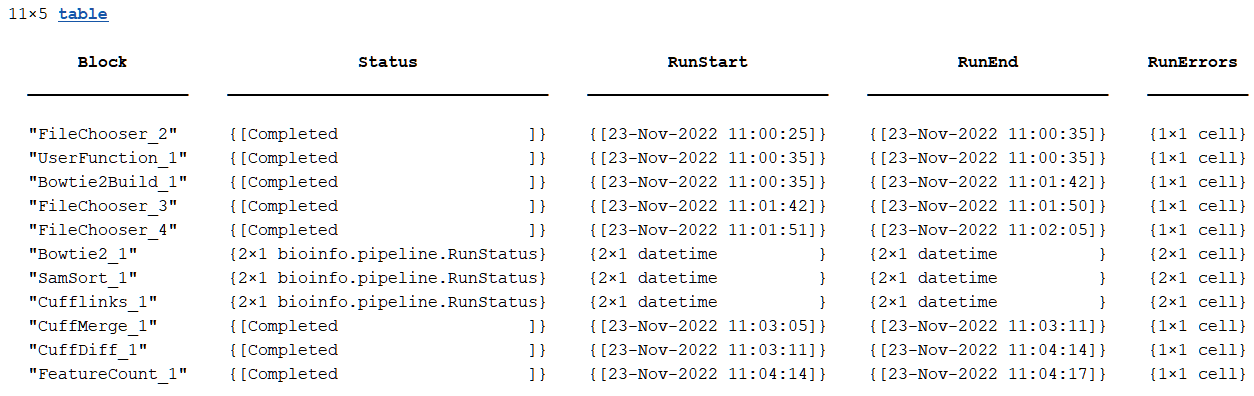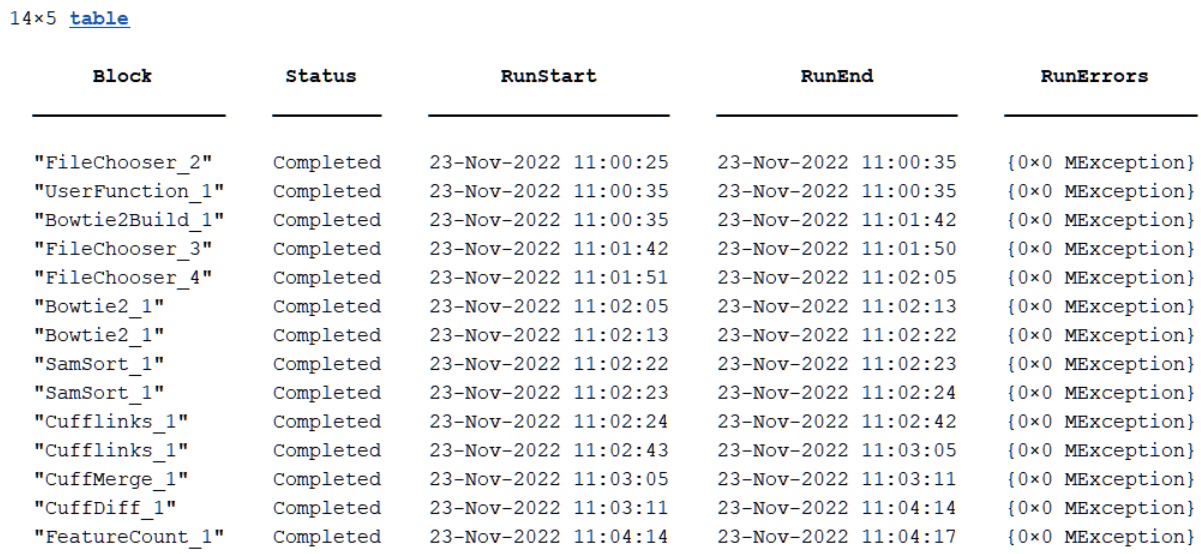# processTable

Return information about all processes in pipeline

Since R2023a

## Syntax

``t = processTable(pipeline)``
``t = processTable(pipeline,blocks)``
``t = processTable(___,Expanded=tf)``

## Description

example

````t = processTable(pipeline)` returns a table `t` that contains information about all processes in a pipeline, including block-specific run start and end times and run statuses.```

example

````t = processTable(pipeline,blocks)` returns a table `t` that contains information only for the specified `blocks`.```
````t = processTable(___,Expanded=tf)` specifies whether to expand `t` to contain one row for each independent run of a block, or to collapse multiple runs of the same block into a single row.```

## Examples

collapse all

Import the pipeline and block objects needed for the example.

```import bioinfo.pipeline.Pipeline import bioinfo.pipeline.block.*```

Create a pipeline.

`P = Pipeline;`

Create a `FileChooser` block that takes in a SAM file as an input.

`samFile = FileChooser(which("Myco_1_1.sam"));`

Create a `SeqTrim` block.

`trimsequences = SeqTrim;`

`addBlock(P,[samFile,trimsequences]);`

Check the names of the output port and input port of the blocks to connect.

`samFile.Outputs`
```ans = struct with fields: Files: [1×1 bioinfo.pipeline.Output] ```
`trimsequences.Inputs`
```ans = struct with fields: FASTQFiles: [1×1 bioinfo.pipeline.Input] ```

Connect the ports.

`connect(P,samFile,trimsequences,["Files","FASTQFiles"]);`

The block returns an incomplete result if the block has not yet run.

`results(P,trimsequences)`
```ans = Incomplete pipeline result. ```

Run the pipeline and check the run status of each block in the process table. The `samFile` block had no error, but the `SeqTrim` block generated an error as indicated by the `Status` column. The `SeqTrim` block generated an error because it was expecting a FASTQ file as an input but received a SAM file instead.

```run(P); t = processTable(P,Expanded=true)```
```t=2×5 table Block Status RunStart RunEnd RunErrors _______________ _________ ____________________ ____________________ ________________ "FileChooser_1" Completed 26-Jul-2023 09:19:07 26-Jul-2023 09:19:07 {0×0 MException} "SeqTrim_1" Error 26-Jul-2023 09:19:07 26-Jul-2023 09:19:08 {1×1 MException} ```

You can see the printed error message at the MATLAB® command line. You can also enter the following commands to see the message.

```seqTrimInfo = t(2,:); seqTrimInfo.RunErrors{:} ```

## Input Arguments

collapse all

Bioinformatics pipeline, specified as a `bioinfo.pipeline.Pipeline` object.

Pipeline blocks, specified as a `bioinfo.pipeline.Block` object, vector of block objects, character vector, string scalar, string vector, or cell array of character vectors representing block names.

Flag to contain one row per each independent block run or group all runs of the same block into a single row, specified as a numeric or logical 1 (`true`) or 0 (`false`).

Each row in the table represents a block, and there are corresponding Status, RunStart, RunEnd, and RunErrors columns for each block.

By default, the function groups (or collapses) all runs of the same block into a single row. If a block is run n independent times (per its `SplitDimension` property of the input port), then the table variable value for each column is an n-by-1 cell array. For instance, the next figure is an example of a (default) collapsed process table (```Expanded = false```). Note that for the Bowtie2_1, SamSort_1 and Cufflinks_1 blocks, the table variable values are 2-by-1 cell arrays because each of these blocks were run 2 times independently.The next figure is the same table with the expanded cell array (```Expanded = true```).## Output Arguments

collapse all

Table containing information on pipeline processes, returned as a table.

Each row in the table represents a block, and there are corresponding Status, RunStart, RunEnd, and RunErrors columns for each block.

## Version History

Introduced in R2023a